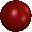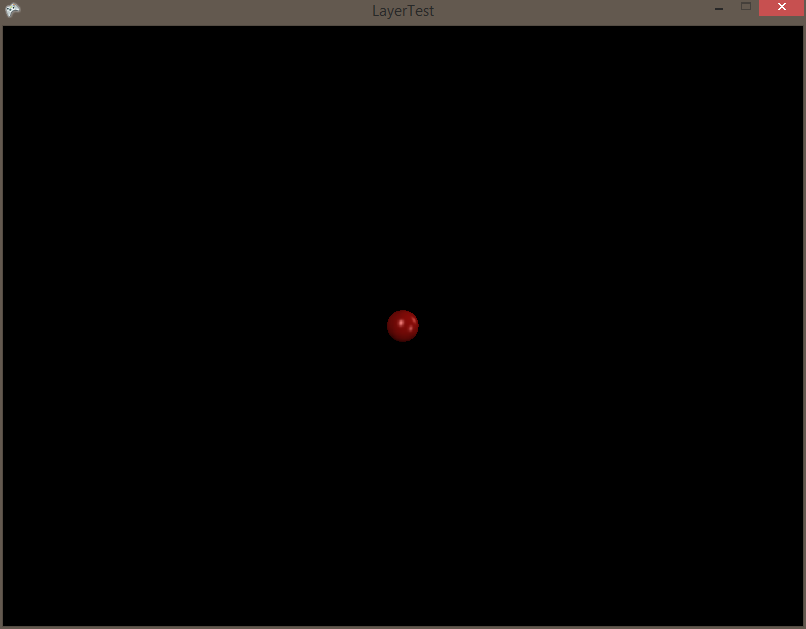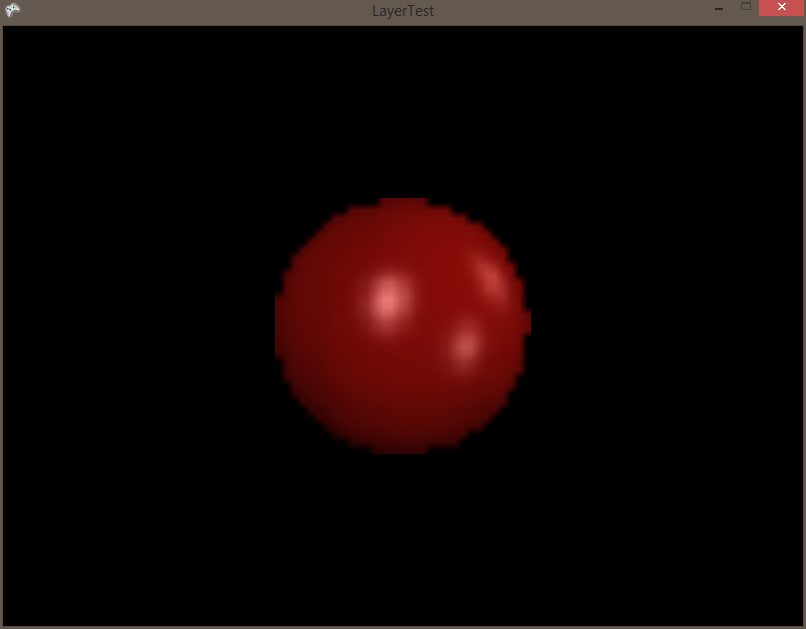# FlatRedBall.Graphics.LayerCameraSettings.OrthogonalWidth

## Introduction

The OrthogonalWidth and OrthogonalHeight of a LayerCameraSettings object controls the number of units wide and high that the Layer will display. The LayerCameraSettings’ Orthogonal value must be true for the OrthogonalWidth and OrthogonalHeight properties to apply.

## Orthogonal Examples

This example was initially constructed in Glue. To reproduce this:

1. Create a Screen
2. Add a Sprite to the Screen
3. Set its Texture (I used)
4. Create a 2D Layer
5. Place the Sprite on the 2D Layer

If you run the game you should now see the Sprite rendering on the Layer:We can now zoom in on this Sprite simply by adjusting the orthogonal values on the LayerCameraSettings. Since I’m using Glue I’ll add the following code to my CustomInitialize for my Screen that contains the Layer:

```// If we want to zoom in 8x, we want to make the amount
// of area that the Layer views 1/8 of what it was before.
// Therefore, we'll divide by 8:
LayerInstance.LayerCameraSettings.OrthogonalWidth /= 8;
LayerInstance.LayerCameraSettings.OrthogonalHeight /= 8;
```

Now the Sprite (and anything on the same Layer) will appear at 8x zoom:## Matching Layer to Camera coordinates

You can match the LayerCameraSettings to match the Camera by setting the OrthogonalWidth/OrthogonalHeight of the LayerCameraSettings to the Camera’s OrthogonalWidth/OrthogonalHeight. This assumes that both the Layer and Camera are using orthogonal values.

```// Assuming layer is a valid Layer
layer.LayerCameraSettings.OrthogonalWidth = Camera.Main.OrthogonalWidth;
layer.LayerCameraSettings.OrthogonalHeight = Camera.Main.OrthogonalHeight;
```

## Using Orthogonal values

Orthogonal values can be used to determine the width and height in world coordinates of the area that a Layer displays. Usually these coordinates happen to match the pixel display area as well, but it is possible for this to not be the case if destination rectangle values are set differently from orthogonal values.

The following code will size a Sprite so that it is the same size as the displayed area of a Layer:

```// Assuming mySprite is a valid Sprite
// Also assuming that myLayer is a valid Layer which has a non-null LayerCameraSettings
// This will be the case if a 2D Layer has been added to a Screen in Glue, and that Screen is active
mySprite.Width = myLayer.LayerCameraSettings.OrthogonalWidth;
mySprite.Height = myLayer.LayerCameraSettings.OrthogonalHeight;
```